Current Electrons in motion make up an electric current. This electric current is usually referred to as “current" or “current flow," no matter how many electrons are moving. Current is a measurement of a rate at which a charge flows through some region of space or a conductor. The moving charges are the free electrons found in conductors, such as copper, silver, aluminum, and gold. The term “free electron" describes a condition in some atoms where the outer electrons are loosely bound to their parent atom. These loosely bound electrons can be easily motivated to move in a given direction when an external source, such as a battery, is applied to the circuit. These electrons are attracted to the positive terminal of the battery, while the negative terminal is the source of the electrons. The greater amount of charge moving through the conductor in a given amount of time translates into a current.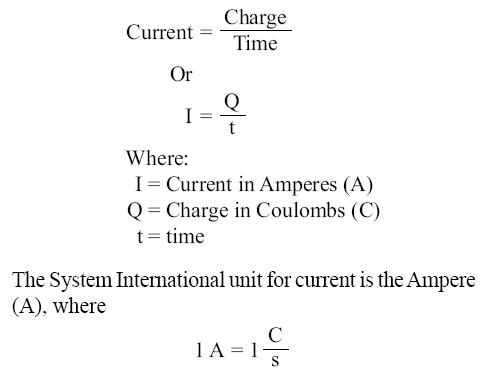That is, 1 ampere (A) of current is equivalent to 1 coulomb (C) of charge passing through a conductor in 1 second(s). One coulomb of charge equals 6.28 billion billion electrons. The symbol used to indicate current in formulas or on schematics is the capital letter “I." When current flow is one direction, it is called direct current (DC). Later in the text, we will discuss the form of current that periodically oscillates back and forth within the circuit. The present discussion will only be concerned with the use of direct current. The velocity of the charge is actually an average velocity and is called drift velocity. To understand the idea of drift velocity, think of a conductor in which the charge carriers are free electrons. These electrons are always in a state of random motion similar to that of gas molecules. When a voltage is applied across the conductor, an electromotive force creates an electric field within the conductor and a current is established. The electrons do not move in a straight direction but undergo repeated collisions with other nearby atoms. These collisions usually knock other free electrons from their atoms, and these electrons move on toward the positive end of the conductor with an average velocity called the drift velocity, which is relatively a slow speed. To understand the nearly instantaneous speed of the effect of the current, it is helpful to visualize a long tube filled with steel balls as shown in Figure 10-37.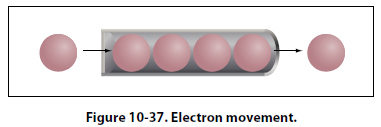It can be seen that a ball introduced in one end of the tube, which represents the conductor, will immediately cause a ball to be emitted at the opposite end of the tube. Thus, electric current can be viewed as instantaneous, even though it is the result of a relatively slow drift of electrons. Ohm’s Law (Resistance) The two fundamental properties of current and voltage are related by a third property known as resistance. In any electrical circuit, when voltage is applied to it, a current will result. The resistance of the conductor will determine the amount of current that flows under the given voltage. In most cases, the greater the circuit resistance, the less the current. If the resistance is reduced, then the current will increase. This relation is linear in nature and is known as Ohm’s law. By having a linearly proportional characteristic, it is meant that if one unit in the relationship increases or decreases by a certain percentage, the other variables in the relationship will increase or decrease by the same percentage. An example would be if the voltage across a resistor is doubled, then the current through the resistor doubles. It should be added that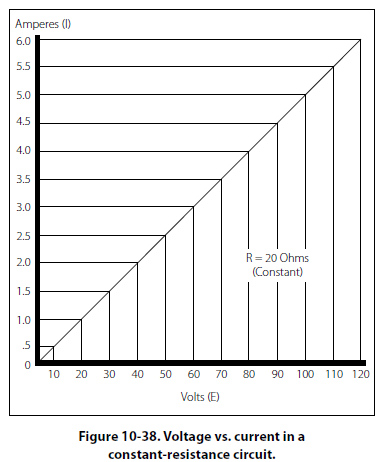this relationship is true only if the resistance in the circuit remains constant. For it can be seen that if the resistance changes, current also changes. A graph of this relationship is shown in Figure 10-38, which uses a constant resistance of 20O. The relationship between voltage and current in this example shows voltage plotted horizontally along the X axis in values from 0 to 120 volts, and the corresponding values of current are plotted vertically in values from 0 to 6.0 amperes along the Y axis. A straight line drawn through all the points where the voltage and current lines meet represents the equation I = E/20 and is called a linear relationship.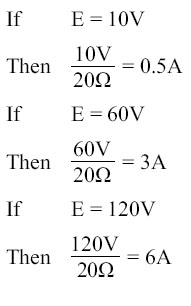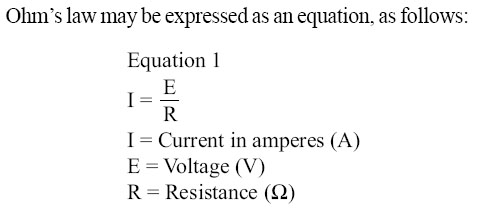Where I is current in amperes, E is the potential difference measured in volts, and R is the resistance measured in ohms. If any two of these circuit quantities are known, the third may be found by simple algebraic transposition. With this equation, we can calculate current in a circuit if the voltage and resistance are known. This same formula can be used to calculate voltage. By multiplying both sides of the equation 1 by R, we get an equivalent form of Ohm’s law, which is: Equation 2 E = I (R) Finally, if we divide equation 2 by I, we will solve for resistance, Equation 3 R = E/I All three formulas presented in this section are equivalent to each other and are simply different ways of expressing Ohm’s law. The various equations, which may be derived by transposing the basic law, can be easily obtained by using the triangles in Figure 10-39.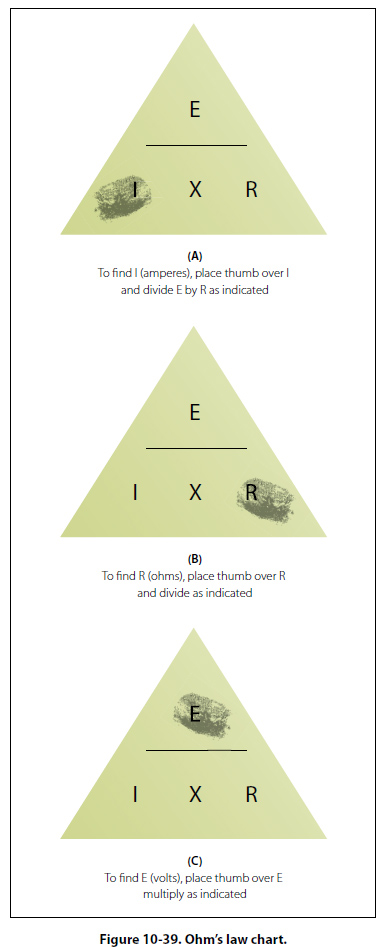The triangles containing E, R, and I are divided into two parts, with E above the line and I × R below it. To determine an unknown circuit quantity when the other two are known, cover the unknown quantity with a thumb. The location of the remaining uncovered letters in the triangle will indicate the mathematical operation to be performed. For example, to find I, refer to Figure 10-39A, and cover I with the thumb. The uncovered letters indicate that E is to be divided by R, or I = E/R. To find R, refer to Figure 10-39B, and cover R with the thumb. The result indicates that E is to be divided by I, or R = E/I. To find E, refer to Figure 10-39C, and cover E with the thumb. The result indicates I is to be multiplied by R, or E = I × R. This chart is useful when learning to use Ohm’s law. It should be used to supplement the beginner’s knowledge of the algebraic method.
 ©AvStop Online Magazine                                                                                                                                                      Contact Us              Return To Books